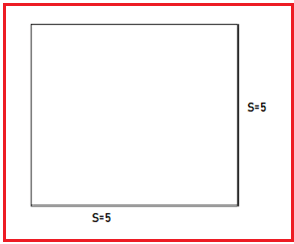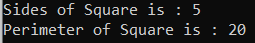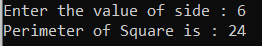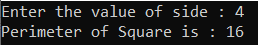# Perimeter of Square in C#

## Calculate Perimeter of Square in C# with Examples

In this article, I am going to discuss How to Calculate the Perimeter of Square in C# with Examples. Please read our previous article, where we discussed how to Calculate the Perimeter of the Rectangle in C#. Here, in this article, first, we will discuss what is Square, then we will discuss how to Calculate the Perimeter of Square and finally, we will see how to implement the Perimeter of Square Program in C#.

##### What is Square?

A Square is a closed, two-dimensional shape. All the sides of a square are equal in length.##### Formula to calculate the perimeter of a square.

The perimeter of a square is the total length of the four sides.
Perimeter = side + side + side + side
Perimeter = 4 * side;

##### Algorithm to Calculate the Perimeter of Square in C#

The following are the steps that we need to follow to solve this problem.
Step1: Take a variable named side and input the length of one of the sides of the square and store it into this.
Step2: Take one more variable named perimeter and store the result of calculation i.e. perimeter = 4 * side;
Step3: Print the value of the perimeter.

##### Example:

The following C# Program will calculate the Perimeter of the Square.

```using System;
public class PerimeterOfSquare
{
public static void Main()
{
decimal side = 5;
decimal perimeter = 4 * side;
Console.WriteLine("Sides of Square is : " + side);
Console.WriteLine("Perimeter of Square is : " + perimeter);
}
}
```
###### Output:##### Example: Taking input from the user

In the following C# program, we will calculate the perimeter of the Square by taking Input from the User.

```using System;
public class PerimeterOfSquare
{
public static void Main()
{
Console.Write("Enter the value of side : ");
decimal perimeter = 4 * side;
Console.Write("Perimeter of Square is : " + perimeter);
}
}
```
###### Output:##### C# Code (Using function)

In the below C# example, we have created one method to calculate the perimeter of Square.

```using System;
public class PerimeterOfSquare
{
public static void Main()
{
Console.Write("Enter the value of side : ");
decimal perimeter = CalculatePerimeterOfSquare(side);
Console.Write("Perimeter of Square is : " + perimeter);